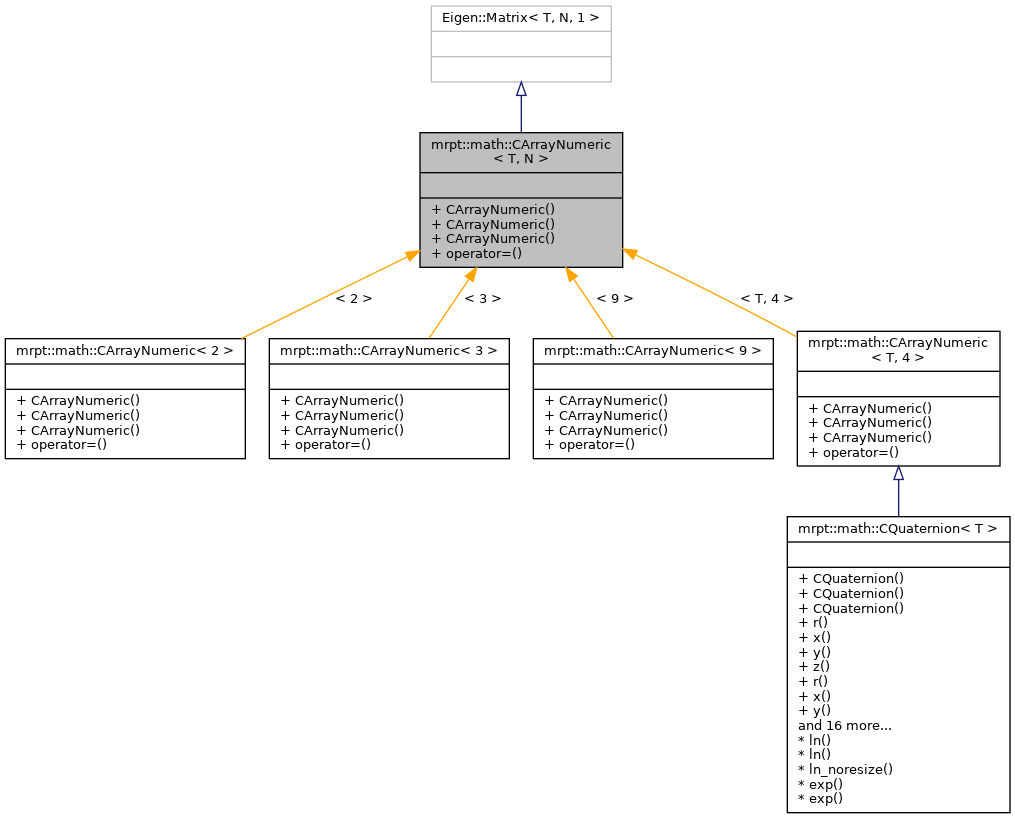MRPT  1.9.9
mrpt::math::CArrayNumeric< T, N > Class Template Reference

## Detailed Description

### template<typename T, std::size_t N> class mrpt::math::CArrayNumeric< T, N >

CArrayNumeric is an array for numeric types supporting several mathematical operations (actually, just a wrapper on Eigen::Matrix<T,N,1>)

CArrayFloat, CArrayDouble, CArray

Definition at line 25 of file CArrayNumeric.h.

`#include <mrpt/math/CArrayNumeric.h>`

Inheritance diagram for mrpt::math::CArrayNumeric< T, N >:## Public Types

using value_type = T

using Base = Eigen::Matrix< T, N, 1 >

## Public Member Functions

CArrayNumeric ()
Default constructor. More...

CArrayNumeric (const T *ptr)
Constructor from initial values ptr-ptr[N-1]. More...

template<class Derived >
CArrayNumeric (const Eigen::MatrixBase< Derived > &obj)
Initialization from a vector-like source, that is, anything implementing operator[]. More...

template<typename OtherDerived >
CArrayNumeric< T, N > & operator= (const Eigen::MatrixBase< OtherDerived > &other)

## ◆ Base

template<typename T, std::size_t N>
 using mrpt::math::CArrayNumeric< T, N >::Base = Eigen::Matrix

Definition at line 29 of file CArrayNumeric.h.

## ◆ value_type

template<typename T, std::size_t N>
 using mrpt::math::CArrayNumeric< T, N >::value_type = T

Definition at line 28 of file CArrayNumeric.h.

## ◆ CArrayNumeric() [1/3]

template<typename T, std::size_t N>
 mrpt::math::CArrayNumeric< T, N >::CArrayNumeric ( )
inline

Default constructor.

Definition at line 32 of file CArrayNumeric.h.

## ◆ CArrayNumeric() [2/3]

template<typename T, std::size_t N>
 mrpt::math::CArrayNumeric< T, N >::CArrayNumeric ( const T * ptr )
inline

Constructor from initial values ptr-ptr[N-1].

Definition at line 34 of file CArrayNumeric.h.

## ◆ CArrayNumeric() [3/3]

template<typename T, std::size_t N>
template<class Derived >
 mrpt::math::CArrayNumeric< T, N >::CArrayNumeric ( const Eigen::MatrixBase< Derived > & obj )
inlineexplicit

Initialization from a vector-like source, that is, anything implementing operator[].

Definition at line 38 of file CArrayNumeric.h.

## ◆ operator=()

template<typename T, std::size_t N>
template<typename OtherDerived >
 CArrayNumeric& mrpt::math::CArrayNumeric< T, N >::operator= ( const Eigen::MatrixBase< OtherDerived > & other )
inline

Definition at line 46 of file CArrayNumeric.h.

 Page generated by Doxygen 1.8.14 for MRPT 1.9.9 Git: 7d5e6d718 Fri Aug 24 01:51:28 2018 +0200 at lun nov 2 08:35:50 CET 2020